# Area of a Trapezoid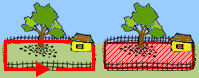The area of a polygon is the number of square units inside that polygon. Area is 2-dimensional like a carpet or an area rug.A trapezoid is a 4-sided figure with one pair of parallel sides. For example, in the diagram to the right, the bases are parallel. To find the area of a trapezoid, take the sum of its bases, multiply the sum by the height of the trapezoid, and then divide the result by 2, The formula looks like this: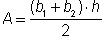orWhereis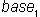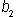is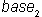is height and · means multiply.

Each base of a trapezoid must be perpendicular to the height. In the diagram above, both bases are sides of the trapezoid. However, since the lateral sides are not perpendicular to either of the bases, a dotted line is drawn to represent the height.

In Examples 1 and 3 below, the height is a side of the trapezoid since it is perpendicular to the base. In Example 2, the lateral sides are not perpendicular to the base, so a dotted line is drawn to represent the height.

Example 1: Find the area of a trapezoid with bases of 10 inches and 14 inches, and a height of 5 inches.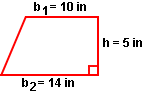Solution:=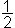· (10 in + 14 in) · 5 in=· (24 in) · (5 in)=· 120 in2= 60 in2

Example 2: Find the area of a trapezoid with bases of 9 centimeters and 7 centimeters, and a height of 3 centimeters.

Solution:=· (9 cm + 7 cm) · 3 cm=· (16 cm) · (3 cm)=· 48 cm2= 24 cm2

Example 3: The area of a trapezoid is 52 square inches and the bases are 11 inches and 15 inches. Find the height.Solution:52 in2 =· (11 in + 15 in) ·52 in2 =· (26 in) ·52 in2 = (13 in) ·52 in2 ÷ (13 in) == 4 in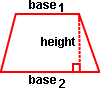Summary: To find the area of a trapezoid, take the sum of its bases, multiply the sum by the height of the trapezoid, and then divide the result by 2, The formula looks like this:orWhereisisis height.

### Exercises

Directions: Read each question below. Click once in an ANSWER BOX and type in your answer; then click ENTER. Your answers should be given as whole numbers greater than zero. After you click ENTER, a message will appear in the RESULTS BOX to indicate whether your answer is correct or incorrect. To start over, click CLEAR.

 1 Find the area of a trapezoid with bases of 5 feet and 7 feet, and a height of 3 feet.  ANSWER BOX:  A =  ft2  RESULTS BOX:
 2 Find the area of a trapezoid with bases of 12 meters and 6 meters, and a height of 5 meters.  ANSWER BOX:  A =  m2  RESULTS BOX:
 3 Find the area of a trapezoid with bases of 17 centimeters and 19 centimeters, and a height of 2 centimeters.  ANSWER BOX:  A =  cm2  RESULTS BOX:
 4 If the trapezoid's area is 48 square inches and the bases are 4 inches and 8 inches. What is the height? ANSWER BOX:  H =  in  RESULTS BOX:
 5 If the trapezoid's area is 77 square centimeters and the bases are 9 centimeters and 13 centimeters. What is the height? ANSWER BOX:  H =  cm  RESULTS BOX: